AsTher Thermodynamic Database and Applications

AsTher Process Calculator for MS Excel

5.5. Example for inverse Calculation using AsTher Process Calculator for MS Excel

A system consists of the elements C, O, H, N.

 Compounds Part. Pressure [bar] CH4 0.09 CO2 0.05 H2O 0.15 H2 0.2 N2 0.5
Soot (carbon) formation is observed.

The average temperature is expected between 300 C and 800 C.

Based on these measurements, the partial pressures for CO- and NOx- as well as the temperature of the system 1 bar will be estimated as follows:

5.5.1. Sheet for gas and solid phases.
Column A: compounds.
Column B: measured partial pressure.
Column C: when a measurement is to use as reference, then latter "x" written in the cells.
Column D is written by application, while the calculation.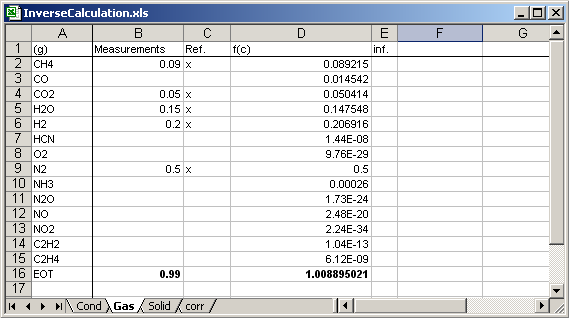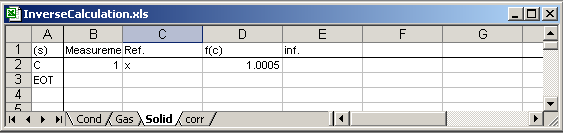5.5.2. Sheet for Conditions
enter in the cell [A1] rcl, the conditions sheet is for inverse calculation (recalculation)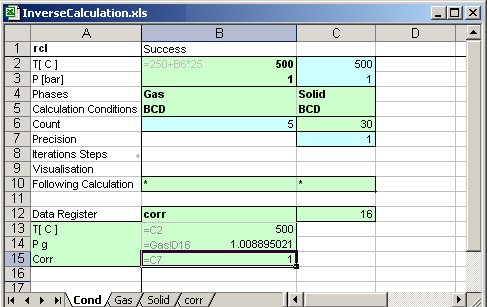B2: Temperature of the thermodynamic system is given by user in depend of the calculations count
B3: Pressure of the thermodynamic system
B4,C4: Phases of the systems, two sheets are created as Gas and Solid.
B5, BCD
First letter B: Column B in the sheet Gas contains measurements
Second letter C: Colmmn C in the sheet Gas contains references
Third letter D: Calculation results are to write in the column D
C5: similar to B5
B6: calculation count is written by application after each calculation
C6: given by user: when B6<C6, then a following calculation executed.

C7: Statistical correlation coefficient of the calculation result written by application.
1: Measurements values are exact consistent.
0:  thermodynamic equilibrium is not possible.

B11: MS Excel file (book) for following calculation '*' mains the same MS Excel book.
B12: Condition sheet of the following calculation, '*' mains the same sheet.
B13: Sheet name, in which the calculation results are to write, the sheet must exists in the same MS Excel book.

Cells [B14] to [B16]: the cells contain links to another cells in a sheet, which data are recorded in the sheet corr.

5.5.3. Calculation

Execute AsTher Process Calculator for MS Excel (XProCalc.exe).
(1) Select as MS Excel file InverseCalculation.xls as MS Excel file
(2) Select as condition sheet Cond
(3) Press button Calculation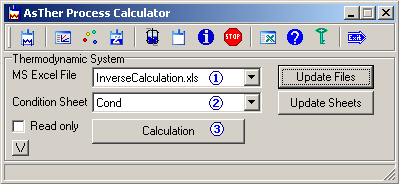5.5.4. Calculation Results
Calculation results are written by application in the columns A, B and C in the sheet corr.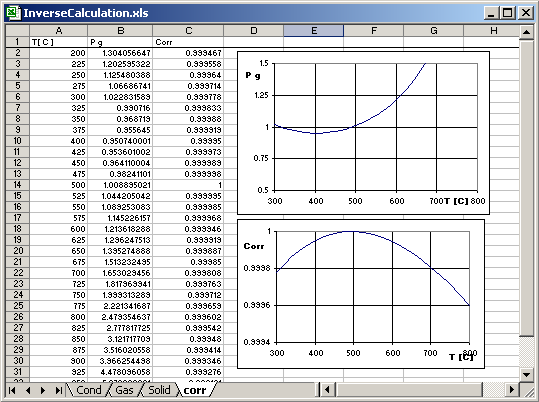Calculations result is 500 C and 1 bar.